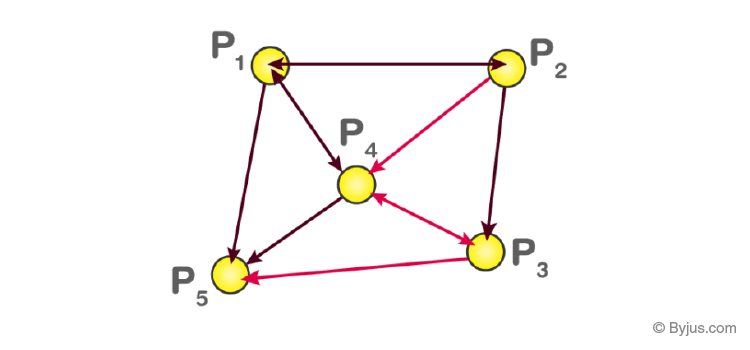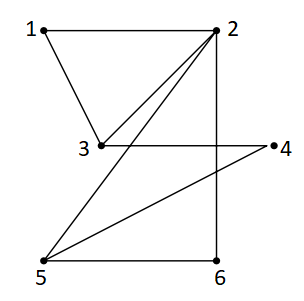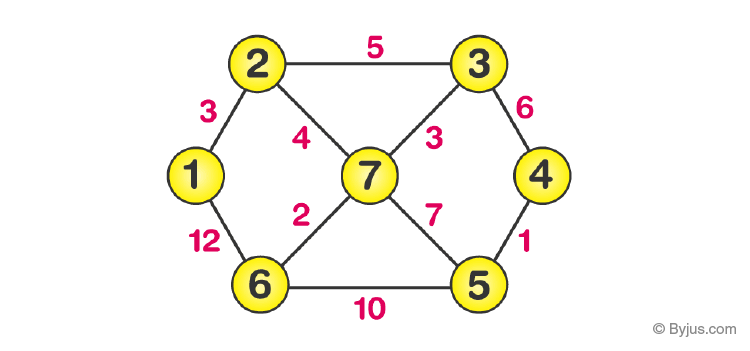In graph theory, an adjacency matrix is nothing but a square matrix utilised to describe a finite graph. The components of the matrix express whether the pairs of a finite set of vertices (also called nodes) are adjacent in the graph or not. In graph representation, the networks are expressed with the help of nodes and edges, where nodes are the vertices and edges are the finite set of ordered pairs.

Graphs can also be defined in the form of matrices. To perform the calculation of paths and cycles in the graphs, matrix representation is used. It is calculated using matrix operations. The two most common representation of the graphs are:

We will discuss here about the matrix, its formation and its properties.

The adjacency matrix, also called the connection matrix, is a matrix containing rows and columns which is used to represent a simple labelled graph, with 0 or 1 in the position of (Vi , Vj) according to the condition whether Vi and Vj are adjacent or not. It is a compact way to represent the finite graph containing n vertices of a m x m matrix M. Sometimes adjacency matrix is also called as vertex matrix and it is defined in the general form as

$$\begin{array}{l}\left\{\begin{matrix} 1 & if \;P_{i}\rightarrow P_{j}\\ 0& otherwise \end{matrix}\right \}\end{array}$$

If the simple graph has no self-loops, Then the vertex matrix should have 0s in the diagonal. It is symmetric for the undirected graph. The connection matrix is considered as a square array where each row represents the out-nodes of a graph and each column represents the in-nodes of a graph. Entry 1 represents that there is an edge between two nodes.

The adjacency matrix for an undirected graph is symmetric. This indicates the value in the ith row and jth column is identical with the value in the jth row and ith column. Additionally, a fascinating fact includes matrix multiplication. If the adjacency matrix is multiplied by itself (matrix multiplication), if there is a nonzero value present in the ith row and jth column, there is a route from Vi to Vof length equal to two. It does not specify the path though there is a path created. The nonzero value indicates the number of distinct paths present.

 Related Links Orthogonal Matrix Matrices Graph Theory Elementary Transformation Of Matrices

## How to create an Adjacency Matrix?

If a graph G with n vertices, then the vertex matrix n x n is given by

$$\begin{array}{l}A=\begin{bmatrix} a_{11} &a_{12} & \cdots &a_{1n} \\ a_{21} &a_{22} &\cdots &a_{2n} \\ \vdots & \vdots &\ddots & \vdots \\ a_{n1}& a_{n2} & \cdots & a_{nn} \end{bmatrix}\end{array}$$

Where, the value aij equals the number of edges from the vertex i to j. For an undirected graph, the value aij = aji for all i, j , so that the adjacency matrix becomes a symmetric matrix.

Mathematically, this can be explained as:

Let G be a graph with vertex set {v1, v2, v3,  . . . , vn}, then the adjacency matrix of G is the n × n matrix that has a 1 in the (i, j)-position if there is an edge from vi to vj in G and a 0 in the (i, j)-position otherwise.

From the given directed graph,  the adjacency matrix is written as$$\begin{array}{l}\text{The adjacency matrix }= \begin{bmatrix} 0 & 1 & 0 & 1 & 1 \\ 1 & 0 & 1 & 1 & 0\\ 0 & 0 & 0 & 1 & 1\\ 1 & 0 & 1 & 0 & 1\\ 0 & 0 & 0 & 0 & 0 \end{bmatrix}\end{array}$$

## Properties

The vertex matrix is an array of numbers which is used to represent the information about the graph. Some of the properties of the graph correspond to the properties of the adjacency matrix, and vice versa. The properties are given as follows:

Matrix Powers

The most well-known approach to get information about the given graph from operations on this matrix is through its powers. The entries of the powers of the matrix give information about paths in the given graph. The theorem is given below to represent the powers of the adjacency matrix.

Theorem: Let us take, A be the connection matrix of a given graph. Then the entries i, j of An counts n-steps walks from vertex i to j.

Spectrum

The study of the eigenvalues of the connection matrix of a graph is clearly defined in spectral graph theory. Assume that, A be the connection matrix of a k-regular graph and v be the all-ones column vector in Rn. Then the i-th entry of Av is equal to the sum of the entries in the ith row of A. This represents the number of edges proceeds from vertex i, which is exactly k.

$$\begin{array}{l}\text{So the } A\vec{v}=\lambda \vec{v} \text{ and this can be expressed as:}\end{array}$$
$$\begin{array}{l}A=\begin{bmatrix} 1\\ 1\\ \vdots \\ 1\end{bmatrix}.\begin{bmatrix} k\\ k\\ \vdots \\ k\end{bmatrix}=k\begin{bmatrix} 1\\ 1\\ \vdots \\ 1\end{bmatrix}\end{array}$$
$$\begin{array}{l}\text{Where } \vec{v} \text{ is an eigenvector of the matrix A containing the eigenvalue k}\end{array}$$

Isomorphisms

The given two graphs are said to be isomorphic if one graph can be obtained from the other by relabeling vertices of another graph. It is noted that the isomorphic graphs need not have the same adjacency matrix. Because this matrix depends on the labelling of the vertices. But the adjacency matrices of the given isomorphic graphs are closely related.

Theorem: Assume that, G and H be the graphs having n vertices with the adjacency matrices A and B. Then G and H are said to be isomorphic if and only if there is an occurrence of permutation matrix P such that B=PAP-1.

For an undirected graph, the protocol followed will depend on the lines and loops. That means each edge (i.e., line) adds 1 to the appropriate cell in the matrix, and each loop adds 2. Thus, using this practice, we can find the degree of a vertex easily just by taking the sum of the values in either its respective row or column in the adjacency matrix. This can be understood using the below example.From this, the adjacency matrix can be shown as:

$$\begin{array}{l}A=\begin{bmatrix} 0 & 1 & 1 & 0 & 0 & 0\\ 1 & 0 & 1 & 0 & 1 & 1\\ 1 & 1 & 0 & 1 & 0 & 0\\ 0 & 0 & 1 & 0 & 1 &0 \\ 0 & 1& 0& 1& 0& 1\\ 0 & 1& 0& 0& 1& 0 \end{bmatrix}\end{array}$$

As explained in the previous section, the directed graph is given as:The adjacency matrix for this type of graph is written using the same conventions that are followed in the earlier examples.

Question:

Write down the adjacency matrix for the given undirected weighted graphSolution:

The weights on the edges of the graph are represented in the entries of the adjacency matrix as follows:

$$\begin{array}{l}A = \begin{bmatrix} 0 & 3 & 0 & 0 & 0 & 12 & 0\\ 3 & 0 & 5 & 0 & 0 & 0 & 4\\ 0 & 5 & 0 & 6 & 0 & 0 & 3\\ 0 & 0 & 6 & 0 & 1 & 0 & 0\\ 0 & 0 & 0 & 1 & 0 & 10 & 7\\ 12 &0 & 0 & 0 & 10 & 0 & 2\\ 0 & 4 & 3 & 0 & 7 & 2 & 0 \end{bmatrix}\end{array}$$

For more such interesting information on adjacency matrix and other matrix related topics, register with BYJU’S -The Learning App and also watch interactive videos to clarify the doubts.